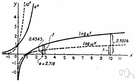# exponential function

(redirected from Natural exponential function)
Also found in: Thesaurus, Encyclopedia.
ThesaurusAntonymsRelated WordsSynonymsLegend:
 Noun 1exponential function - a function in which an independent variable appears as an exponentexponentialfunction, mapping, mathematical function, single-valued function, map - (mathematics) a mathematical relation such that each element of a given set (the domain of the function) is associated with an element of another set (the range of the function)
Site: Follow: Share:
Open / Close